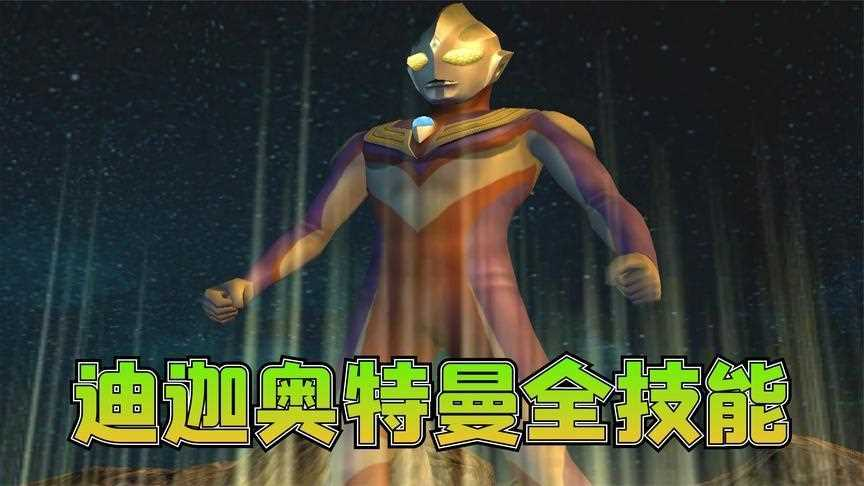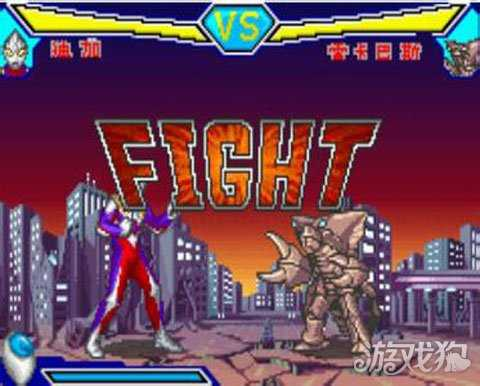DVG游戏网，好玩的手机游戏推荐！

# 《奥特曼格斗版》出招表按键大全A：踢 B：拳

-=超人篇=-

ULTRAMAN

↙+B(上钩拳)

A+L(发射激光束)

A+R(向空中发射两束能量波)

B+L(水平方向投掷道具)

B+R(向空中投掷道具)

ULTRASEVEN

A+L(发射激光束)

↓+A+L(发射细激光束，略低)

B+L(冲撞)

ULTRAMANIACK

↘+A(滑步)

A+L(发射激光束)

↓+A+L(发射细激光束，略低)

B+L(投剑)

B+R(向空中开火)

↓+B+R(将力量注入大地，然后力量从一定距离的地面飞出)

ULTRAMANTABO

↘+B(重拳攻击)

A+L(发射激光束，威力巨大)

B+L(全身燃起火焰，奔跑攻击，击中对手后，剧烈爆炸，威力巨大)

ULTRAMANTIGA

↘+A(2 HITS踢)

B+L(变身，初态(蓝红相间)

B+R(变身，初态(蓝红相间)

A+L(发射激光束)

A+R(瞬间移动)

A+L(能量投射)

A+R(翻腾夹摔)

A+L(电光投掷)

A+R(身体压制)

ULTRAMANDYNA

B+L(变身，初态(蓝红相间)

B+R(变身，初态(蓝红相间)

A+L(发射激光束)

A+R(向空中投掷道具)

A+L(超重拳攻击)

A+R(空中重踢)

A+L(火光投射)

A+R(翻滚攻击)

ULTRAMANGAIA

↘+A(滑步)

A+L(能量投射)

A+R(冲刺拳)

B+L(投掷大道具，速度慢)

B+R(投掷小道具，速度快)

ULTRAMANAGUI

→+A(踢到空中，可接A)

A+L(发射激光束)

A+R(发射大能量球)

B+L(剑刺)

B+R(发射小能量球)

-=怪物篇=-

ALIEN RAITAN

→→/←←空中可

A+L(开火，一次一个)

B+L(开火，一次三个)

DINO-TANK

A+L(发射激光)

B+L(向空中开火)

Z-TON

A+L(发射火球)

B+L(向地面扫射)

TYRANT

A+L(链子攻击，可将对手拉过来)

B+L(喷火)

GATANOTHOR

A+L(触手攻击，右方)

B+L(触手攻击，左方)

REICUBAS

A+L(喷出气体)

B+L(开火)

#### 阅读排行

Copyright 2020-2030 www.dvg.cn 【DVG游戏网】 版权所有 蜀ICP备20010799号-1